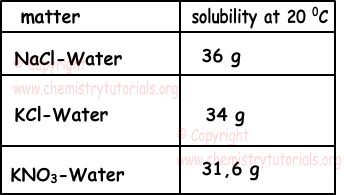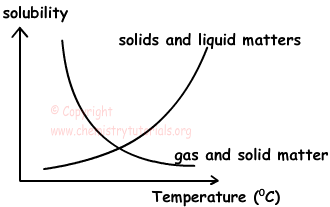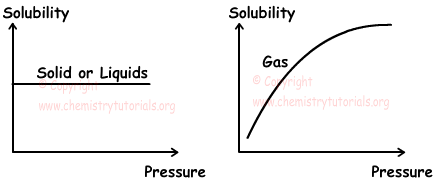EN | ES | DE | NL | RU

## Solubility and Factors Affecting Solubility

Solubility and Factors Affecting Solubility

Solubility is the amount of solute in 100 cm3 (100 mL) solvent.

Example: In 100 g water at 20 0C, 36 g salt can be dissolved. Thus solubility of salt at 20 0C 100 g water is 36g/100g

Solubility is characteristic property of matters, we can distinguish matters by knowing their solubility values at same temperature. Table given below shows solubility of some matters at 20 0C;Example: 25 g X salt is put into 40 cm3 water at 20 0C. After dissolution process, 15 g X stays undissolved at the bottom of the tank. Find solubility of X at 20 0C 100 g water.

Solution:

25-15=10 g X dissolves in 40 cm3 water.

40 cm3 water dissolves 10 g X

100 cm3 water dissolves ? g X

¯¯¯¯¯¯¯¯¯¯¯¯¯¯¯¯¯¯¯¯¯¯¯¯¯¯

?=25 g X

Solubility of X in 100 g water at 20 0C is 25g/100 cm3

Example: If solubility of KCl in water at room temperature is 25g/100cm3, which ones of the following solutions are saturated.

I. 50 g water - 15 g KCl

II. 30 g water - 10 g KCl

III. 20 g water - 3 g KCl.

Solution:

I. 100 g water dissolves 25 g KCl

50 g water dissolves X g KCl

¯¯¯¯¯¯¯¯¯¯¯¯¯¯¯¯¯¯¯¯¯¯¯¯¯¯

X=12,5 g KCl dissolves. Thus, 15-12,5=2,5 g KCl stays undissolved at the bottom of tank.

II. 100 g water dissolves 25 g KCl

30 g water dissolves X g KCl

¯¯¯¯¯¯¯¯¯¯¯¯¯¯¯¯¯¯¯¯¯¯¯¯¯¯

X=7,5 g KCl dissolves. Thus, 10-7,5=2,5 g KCl stays undissolved at the bottom of tank.

III. 100 g water dissolves 25 g KCl

20 g water dissolves X g KCl

¯¯¯¯¯¯¯¯¯¯¯¯¯¯¯¯¯¯¯¯¯¯¯¯¯¯

X=5 g KCl dissolves. Thus, if we add  5-3=2 g KCl it can also be dissolved in 20 g water.

Thus, I and II are saturated solutions and III is unsaturated solution.

Factors Affecting Solubility

Solvent and types of solute, temperature, pressure and common ion effect are factors affecting solubility.

1) Solvent and Types of Solute:

• If molecular structures of solute and solvent are similar, more solute are dissolved in solvent with respect to solutions having dissimilar solute and solvent molecule structure.
• Polarmatterslikeacids, bases, salts, alcohol and sugar are very soluble in polar solvents like water.
• Nonpolar matters like I2, Br2 are very soluble in nonpolar matters like CCl4.

2) Temperature:

Some of the matters dissolve better by increasing temperature, on the contrary some of them dissolve better by decreasing temperature. Solutions taking heat are called endothermic solutions and solutions giving heat are called exothermic solutions.

a) Endothermic Solutions: Most of the solids need heat to dissolve like;

X(s) + Heat → X(aq)

In this type of solutions, solubility increases with increasing temperature.

a) Exothermic Solutions: Most of the gases give heat to dissolve like;

Y(g)  → Y(aq) + Heat

In this type of solutions, solubility decreases with increasing temperature.Example: Look at following reactions and find which ones of them have solubility increasing with temperature.

I. XY(s) + Heat → X+2(aq) + Y-2

II. XY2(s) → X+2(aq) + 2Y-1(aq) + Heat

III. XY3(s) → X+3(aq) + 3Y-1(aq) + Heat

Solution: In endothermic solutions, solubility increases with increasing temperature. Thus, since I. is endothermic reaction solubility of it increases with increasing temperature. II and III are exothermic reactions, so solubility of them decreases with temperature.

3) Pressure:

Pressure changes only solubility of gases in liquids. Solubility of gases in liquids increases with increasing partial pressure and decreases with decreasing partial pressure.4) Common Ion:

Solubility of any solid matter having common ions with solvent is lower than solubility in pure solvents. For example, solubility of AgNO3 in pure water is larger than solubility of AgNO3 in NaNO3 since they have common ion NO3-.

Example: Compare solubility of NaCl in following solvents;

I. Pure water

II. NaNO3(aq)

III. Na2SO4(aq)

Solubility of NaCl in pure water is larger than others since they have no common ions. NaCl has one common ion with NaNO3 and 2 common ion with Na2SO4. Increasing in the number of common ion decreases solubility. Thus;

I>II>III

Factors Effecting Speed of Solvation

• Types of matter
• Changing temperature ( decreasing for exothermic solutions and increasing for endothermic solutions)
• Surface of contact (granulated sugar dissolves faster than cube sugar)
• Mixing solution increases speed of solvation.

Solutions Exams and  Problem Solutions

The Original Author: